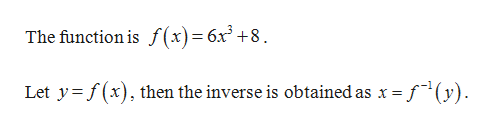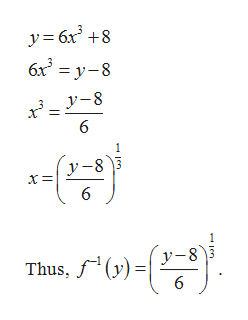What is the inverse of f(x) =6x^3+8 ?

Question

What is the inverse of f(x) =6x^3+8 ?

Step 1

Given:help_outlineImage TranscriptioncloseThe function is f(x)= 6x+8 Let y f(x), then the inverse is obtained as x = . (y) fullscreen
Step 2

Obtain the inverse of the f...help_outlineImage Transcriptionclosey 6x3 8 6x3 = y-8 6 y-8 3 y-8 Thus, y) 6 en fullscreen

Want to see the full answer?

See Solution

Want to see this answer and more?

Our solutions are written by experts, many with advanced degrees, and available 24/7

See Solution
Tagged in

Math